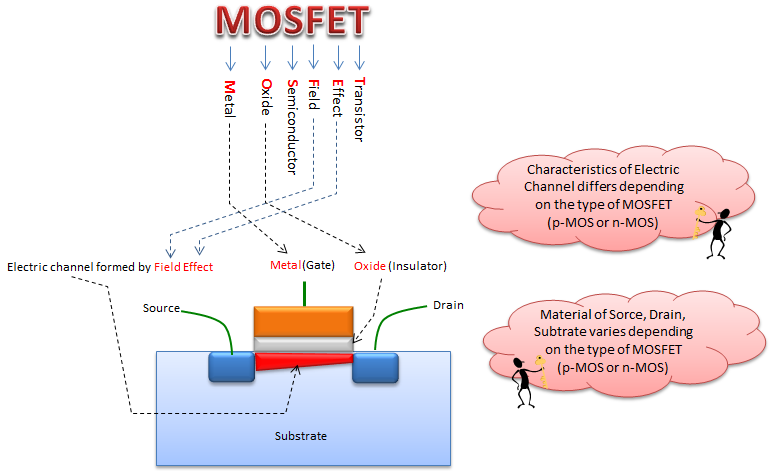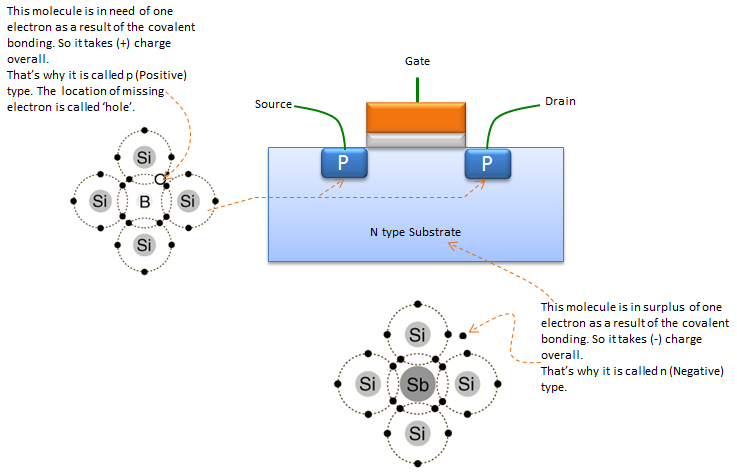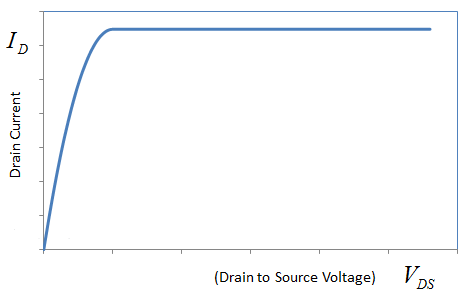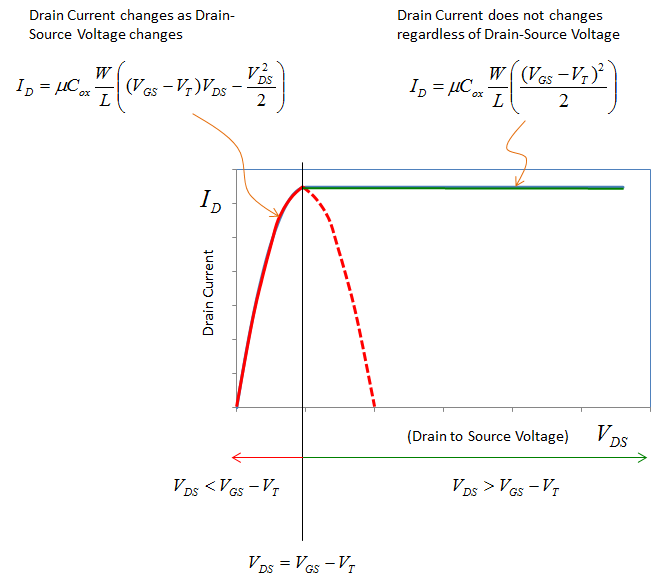Electronics - MOSFET                                             Home : www.sharetechnote.com MOSFET stands for Metal Oxide Semiconductor Field Effect Transiter. The term itself represents the fundamental structure of the device itself as shown below.Molecular Structure of MOSFET   Molecular structure of the MOSFET can be illustrated as follows. The specific elements being used in each substrate can change depending on the specific type of the MOSFET, but general physical properties of the substrate is same as shown here. That is, in P substrate we have element (atom) that forms covalent bond that create hole and in N substrate we have an element (atom) that forms covalent bond that create free electron.Mathematical Modeling   If you measure the I-V characteristics of MOSFET, it would be some as shown below. How can we represent this curve in mathematical form.There can be several different ways to represent this curve in a mathematical form. Some can represent the curve in a single expression and others would represent the curve in a combination of multiple expressions. In this section, I would show you a form that represent the curve with a combination of two mathematical expressions. In this method, we split the curve into two parts as shown below, the solid red line part and the solid green line. If you look closely at the red solid line part, you may intuitively think 'This looks like a left side of a quadratic function. This quadratic function would look like the red solid line and red dotted line'. The equation of this quadratic function is as shown below. Does it look too complicated ? Don't be scared. It is the exactly same form of y = a x^2 + b that you learned in high school. The only differences are y is replaced with I_D and x is replaced with V_DS and all the others are just a constant that will be given to you). Now let's look at the solid green line. It may look like a horizontal line which means a constant function. It means this is a line with zero slope. It can be expressed in the form of y = c where c is a constant. y is replaced with I_D here and all other parameters will be given to you as a constant.Why MOSFET ?   Why the MOSFET is so popular ? Any Advantage of MOSFET over conventional JFET ?   Because the gate is insulated electrically from the channel, no current flows between the gate and the channel, no matter what the gate voltage (However if you put too high gate voltage, it would physically breakdown the metallic oxide layer). Thus, the MOSFET has practically infinite impedance . This makes MOSFETs useful for power amplifiers. The devices are also well suited to high-speed switching applications.     Any Disadvantage ?   Because the oxide layer is so thin, the MOSFET is susceptible to permanent damage by electrostatic charges. Even a small electrostatic buildup can destroy a MOSFET permanently. In weak-signal radio-frequency(RF), MOSFET devices do not generally perform as well as other types of FET. (This issue with RF can be solved by using GaAsFET)